Grade 2 Maths - Common Core This group is for Grade 2 Mathematics. The sub-topics for this group is based on Common Core Mathematics Standards � Grade 2 : http://www.corestandards.org/Math/Content/2/introduction/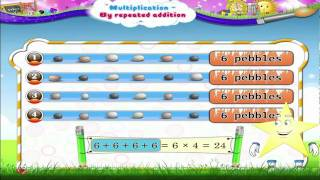MultiplicationLearn Grade 2 - Maths - Multiplication by Repeated AdditionLearn Grade 2 - Maths - Multiplication by Repeated Addition.This video follows Robert Blitzers Introductory and Intermediate Algebra for College Students text ...07-Jun-2015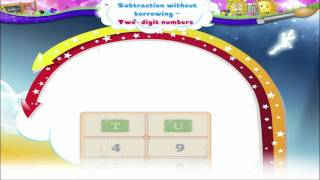add and subtract within 100Learn Grade 2 - Maths - Subtraction without Borrowing Two Digit NumbersIn this video you get to Learn Grade 2 - Maths - Subtraction without Borrowing Two Digit Numbers.06-Jun-2015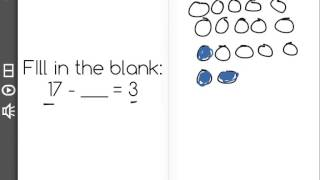add and subtract within 100[2.MD.5-1.6] Add/Subtract within 100 - Common Core Standard - Practice ProblemDiscover more Common Core Math at https://www.frontrowed.com Understand that addition and subtraction are inverse operations (4 + ___ = 10 and 10 - 4 ...06-Jun-2015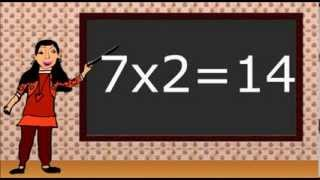MultiplicationMath. Multiplying. For 2nd and 3rd grade.Math for second and third grade. Multiplying by 0,1,2,3. Multiplication flash cards.12-May-2015Add and subtract within 20Add and subtract (word problems 20 max) - 2nd GradeThis video covers addition and subtraction word problems up to the number 20 at the 2nd Grade level. It includes a short lesson and the worked out answers to ...12-May-2015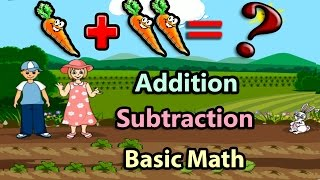Add and subtract within 20Basic Math For Kids: Addition and Subtraction, Science games, Preschool and Kindergarten ActivitiesAnnie and Jose have fun with Addition and Subtraction in their new garden. Addition and subtraction are the most basic things of Mathematics. In this basic math ...12-May-2015Solve problems involving addition and subtractionSubtraction Problem Solving Using Model Math Grade 2In this video, Mrs. Jousan models the step by step problem solving approach based on the Singapore Model Math involving addition. This is aligned to the ...12-May-2015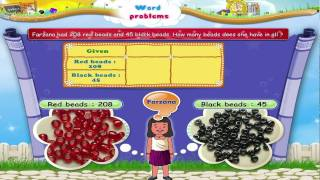Solve problems involving addition and subtractionLearn Grade 3 - Maths - Addition and Subtraction Word ProblemsIn this video you get to Learn Grade 3 - Maths - Addition and Subtraction Word Problems.12-May-2015
Search Results fromNext>>